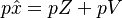# Leontief Price Model

## Definition

Leontief Price Model (also cost-push IO model) denotes an input-output system, where prices are determined from a set of equations which states that the price which each sector of the economy receives per unit of output must equal the total outlays incurred in the course of its production. 

The outlays comprise not only payments for inputs purchased from the same and from other industries as well as imports but also the GVA, which essentially represents payments made to the external factors, for example, capital, labour, and land including residual profits.

## Formula

The essence of the Leontief price model lies in the causal relationships between these prices. The primary input prices (equal per IO row) are assumed to be exogenous, whereas the prices for total output (also equal per IO row) are endogenously determined by the solution of$p \hat{x} = p Z + p V$

where:

• p = I-vector with (index)prices for total sectoral output,
• p v = P-vector with (index)prices for primary inputs per category.